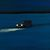# Arcade: Snap point to polyline (mid-segment)

450
2
03-19-2022 08:28 AM
Status: Open
Labels (1)by
Frequent Contributor II

Snap a point to a polyline — but snap it mid-segment, not just to the closest vertex.

The function would return the snapped point — as well as return the ID of the polyline the point was snapped to.Thanks.

Tags (1)byYes, a snap function would be great.

In the meantime, you can do it yourself (this is in multiple of my Calculation Attribute Rules):

``````function closest_feature(test_feature, compare_feature_set) {
// returns the feature of compare_feature_set that is closest to test_feature
var min_distance = 9999999
var closest_feature = null
for(var f in compare_feature_set) {
var d = Distance(test_feature, f)
if(d < min_distance) {
min_distance = d
closest_feature = f
}
}
return closest_feature
}

function project_orthogonally(point_geometry, line_geometry) {
// returns a projection of the point_geometry onto the line_geometry
// only start and end point of the line_geometry are considered!
// https://de.wikipedia.org/wiki/Orthogonalprojektion
// https://en.wikibooks.org/wiki/Linear_Algebra/Orthogonal_Projection_Onto_a_Line
var p = point_geometry
var r0 = line_geometry.paths
var r1 = line_geometry.paths[-1]
var ux = r1.x - r0.x
var uy = r1.y - r0.y
var lambda = ((p.x-r0.x)*ux + (p.y-r0.y)*uy) / (ux*ux + uy*uy)
var new_p = Point({"x": r0.x + lambda * ux, "y": r0.y + lambda * uy, "spatialReference": p.spatialReference})
// if new_p is on the line defined by r0 and r1 but not on the actual line_geometry, snap it to the closest end point
if(Disjoint(new_p, line_geometry)) {
new_p = IIF(Distance(r0, p) < Distance(r1, p), r0, r1)
}
return new_p
}

function snap_point_to_polyline(point_geometry, polyline_geometry) {
// returns a point that is snapped to the polyline_geometry

// create feature set of the polyline's segments
var sr = point_geometry.spatialReference
var vertices = polyline_geometry.paths
var fs_segments = {"fields": [], "spatialReference": sr, "geometryType": "esriGeometryPolyline", "features": []}
for(var s=0; s<Count(vertices)-1; s++) {
var p0 = vertices[s]
var p1 = vertices[s+1]
Push(fs_segments.features, {"geometry": {"paths": [[ [p0.x, p0.y], [p1.x, p1.y] ]], "spatialReference": sr}})
}
fs_segments = FeatureSet(Text(fs_segments))
// project point_geometry onto the closest segment
var closest_segment_geo = Geometry(closest_feature(point_geometry, fs_segments))
return project_orthogonally(point_geometry, closest_segment_geo )
}

var fs_lines = FeatureSetByName(\$datastore, "Lines")
// optionally, buffer
fs_lines = Intersects(fs_lines, Buffer(\$feature, 100))

var closest_line_geometry = Geometry(closest_feature(\$feature, fs_lines))
return snap_point_to_polyline(Geometry(\$feature), closest_line_geometry)``````by

Related:

Attribute Rules Blog: Auto Snapping with Attribute rules during Editing